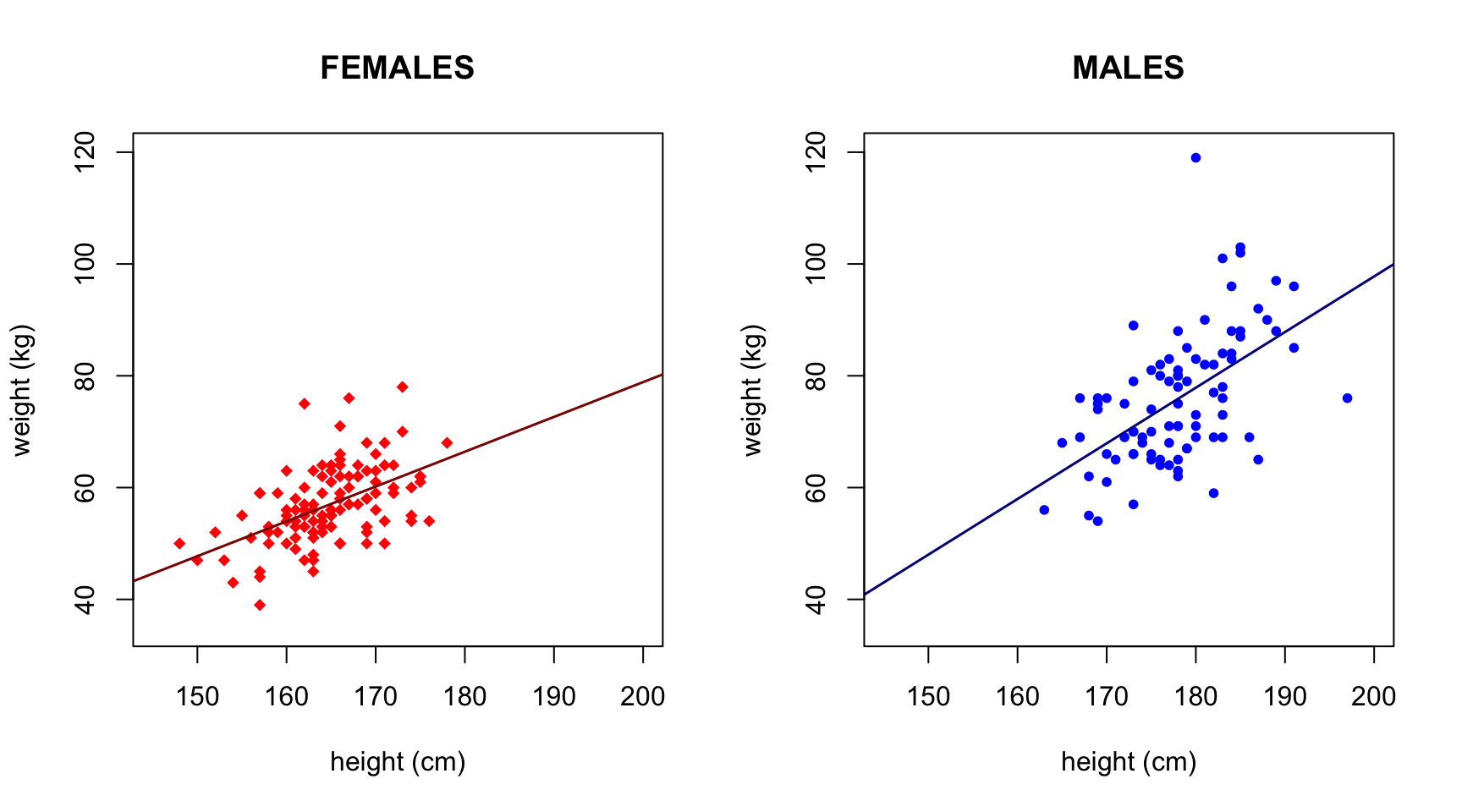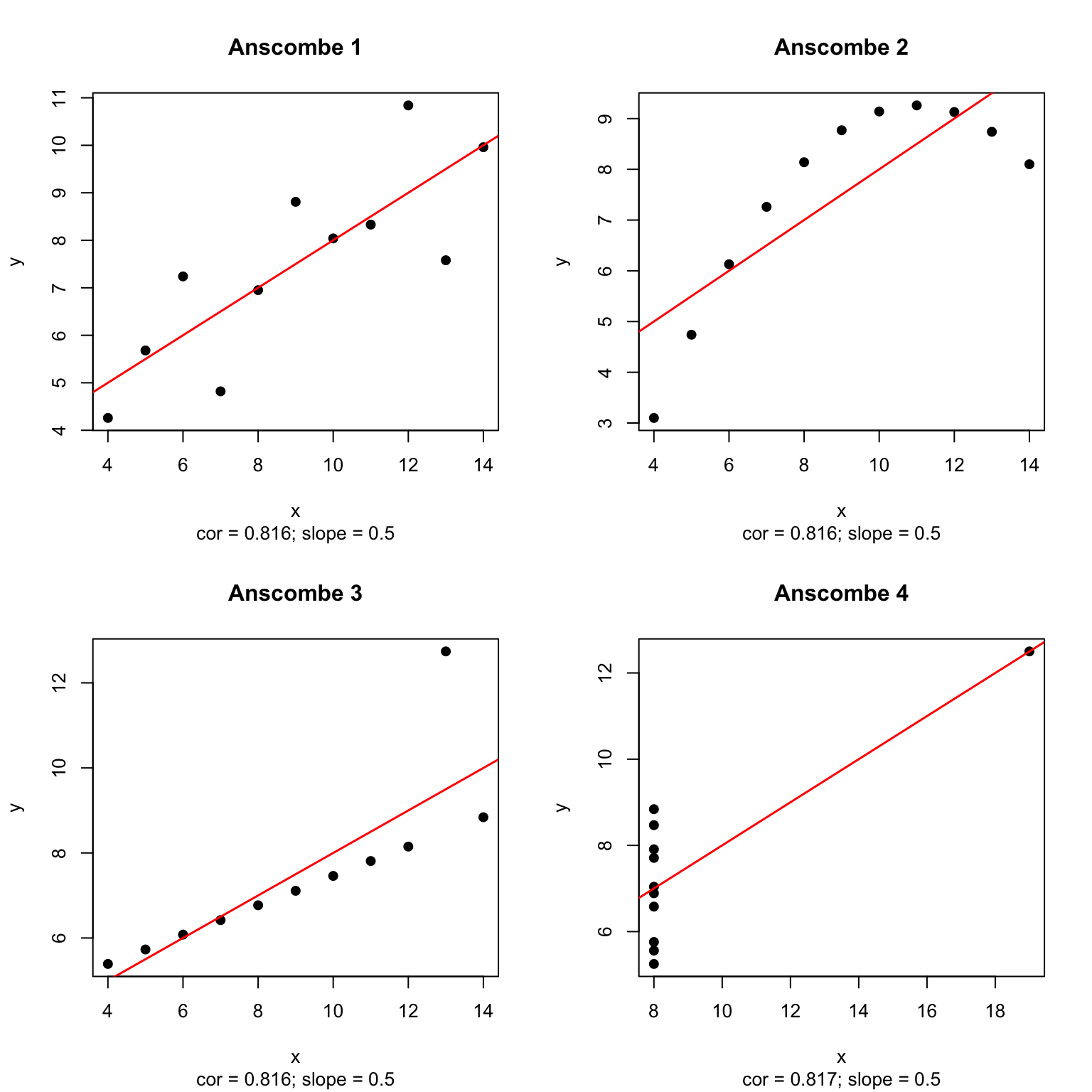In this lecture, we will study more details about the linear regression model. In particular, how to make predictions from the model using R, and how to assess whether the model seems approriate for the data set at hand. Finally, we will study the interpretation of the coefficients in multiple regression setting where many predictors are simultaneously included in the model.

Let’s start by reading in the same height-weight data set as previously.

measures = read.table("Davis_height_weight.txt", as.is = TRUE, header = TRUE)
head(measures, n = 3) #show first three rows
##   X sex weight height repwt repht
## 1 1   M     77    182    77   180
## 2 2   F     58    161    51   159
## 3 3   F     53    161    54   158

The columns repwtand repht are self-reported weight and self-reported height, respectively.

Let’s fit separate linear regression models lm.m and lm.f in males and females, respectively.

ii.m = (measures$sex == "M") #logical vector with 'TRUE' for Males and 'FALSE' for Females lm.m = lm( weight ~ height, data = measures[ii.m,]) #fit model in males lm.f = lm( weight ~ height, data = measures[!ii.m,]) #fit model in females #use same range on the axes in both sexes to get a clear picture how they compare x.range = c(145, 200) #height in cm y.range = c(35, 120) #weight in kg x.name = "height (cm)" y.name = "weight (kg)" par(mfrow = c(1,2)) #split plotting area to 1 x 2 grid plot(measures$height[!ii.m], measures$weight[!ii.m], pch = 18, main = "FEMALES", col = "red", ylab = y.name, xlab = x.name, xlim = x.range, ylim = y.range) abline(lm.f, col = "darkred", lwd = 1.5) #add the regression line plot(measures$height[ii.m], measures$weight[ii.m], pch = 20, main = "MALES", col = "blue", ylab = y.name, xlab = x.name, xlim = x.range, ylim = y.range) abline(lm.m, col = "darkblue", lwd = 1.5) #add the regression line### Predictions from the regression model In the previous Lecture, we looked up the coefficients of the linear model and used them to compute the predicted value of weight for an individual with a given height. For example, to compute the predicted weight for a 180 cm tall male, we did sum(lm.m$coeff*c(1,180)) #this computes a*1 + b*180 for a and b taken from male regression 'lm.m'
##  77.87761

Very generally, in R, the fitted models can be used for prediction via the predict(model, newdata) function. Let’s predict weight for two new individuals who are 160 cm and 180 cm tall. We need to give the input data as parameter newdata = formatted as a data.frame that contains (at least) the same column names that were used in the model fitted by the lm() function. In the current case, we need to define only the column height. We will call the data that goes in to the prediction as data.in.

data.in = data.frame(height = c(160, 180))
data.in # Check that this looks correct.
##   height
## 1    160
## 2    180
predict(lm.m, newdata = data.in) # predictions for males of 160cm and 180cm
##        1        2
## 57.96565 77.87761

We can present these results in a data.frame together with the input data:

data.frame(data.in, predicted.weight = predict(lm.m, newdata = data.in))
##   height predicted.weight
## 1    160         57.96565
## 2    180         77.87761

The uncertainty of prediction can be asked at the level of individual value interval = "prediction" or as a confidence interval for the population mean at the given parameter values interval = "confidence". Note that the option names in R commands can be abbreviated as long as the abbreviation remains unique among all possible options; hence here we can use pred and conf, for example.

predict(lm.m, newdata = data.in, interval = "pred") #for males of 160cm and 180cm
##        fit      lwr      upr
## 1 57.96565 36.95574 78.97555
## 2 77.87761 57.73251 98.02271

The interpretation of the 2nd prediction interval here is that, in 95% of the data sets, when we do this prediction procedure for a new male who is 180 cm tall, the new male will fall within the prediction interval, which here is from 58 kg to 98 kg.

predict(lm.m, newdata = data.in, interval = "conf") #for males of 160cm and 180cm
##        fit      lwr      upr
## 1 57.96565 51.59499 64.33631
## 2 77.87761 75.64286 80.11236

The interpretation of the confidence interval for the mean here is that, in 95% of the data sets, when we do this prediction procedure for male population of height of 180 cm, the true population mean will be within the confidence interval, which here is from 75.6 kg to 81.1 kg.

WARNING: A common error happens when newdata parameter is not correctly specified because then predict() will not complain but simply outputs the fitted values of the original lm() model fit. For example, if we misspell newdata as new.data, R will not complain and the first two predicted values are actually for the first 2 males in the original data, NOT for 160cm and 180cm as the user might think!

predict(lm.m, new.data = data.in)[1:2] #GOES WRONG because newdata has not been specified!
##        1        4
## 79.86881 74.89082
predict(lm.m, newdata = data.in)[1:2] #This is correct
##        1        2
## 57.96565 77.87761

#### Example 7.1

1. What is the predicted weight of a male who is 170 cm tall? What is the predicted population average weight of all males who are 170 cm tall? Give appropriate 95% intervals in both cases.
#For completeness, let's re-fit the model first in males only
lm.m = lm( weight ~ height, data = measures[measures$sex == "M",]) #predicted weight for a male 170 cm predict(lm.m, newdata = data.frame(height = c(170)), interval = "pred") ## fit lwr upr ## 1 67.92163 47.61119 88.23207 #predicted population average at 170 cm predict(lm.m, newdata = data.frame(height = c(170)), interval = "conf") ## fit lwr upr ## 1 67.92163 64.50355 71.3397 We estimate that the population average weight for a male who is 170 cm tall is 67.9 kg (95%CI: 64.5,…,71.3). We predict that 95% CI for weight values for 170 cm males is (47.6,…,88.2) (and the mean weight is the same 67.9 kg as the estimate for the population mean). 1. Fit a linear model regressing height on weight in females. What is the predicted height of a woman who weighs 60kg? lm.h.on.w = lm( height ~ weight, data = measures[measures$sex == "F",])
#predicted weight for a female of 60kg
predict(lm.h.on.w, newdata = data.frame(weight = c(60)), interval = "pred")
##        fit      lwr      upr
## 1 166.0199 156.2594 175.7805

Predicted height is 166 cm with 95%CI of (156,…,176).

### Assessing model fit

Francis Anscombe generated four data sets of 11 observations that had similar summary statistics (means, variances, correlations) but look very different when plotted. His point was to emphasize the importance to plot the data. In R, these data sets are in the columns of the data.frame called anscombe. Let’s plot the datasets, fit linear model and compute correlations and slopes that we put as a subtitle for each plot.

anscombe[1:2,] # show first two rows 
##   x1 x2 x3 x4   y1   y2   y3   y4
## 1 10 10 10  8 8.04 9.14 7.46 6.58
## 2  8  8  8  8 6.95 8.14 6.77 5.76
par(mfrow = c(2,2)) #2 x 2 plotting area for 4 plots
for(ii in 1:4){
x = anscombe[,ii] #x-coordinates for Anscombe's data set ii
y = anscombe[,4 + ii] #y-coordinates for Anscombe's data set ii
lm.fit = lm(y ~ x)
plot(x, y, main = paste("Anscombe",ii), pch = 19, xlab = "x", ylab = "y",
sub = paste0("cor = ",signif(cor(x,y),3),
"; slope = ",signif(coefficients(lm.fit),3)))
abline(lm.fit, col = "red", lwd = 1.5)
}We see that the data sets are identical with respect to the x-y correlation and the linear model slope, but we also see that the data sets are very different in other respects, and, in particular, the linear model does not seem an appropriate description of the X-Y relationship for the data sets 2, 3 and 4.

How can we know whether a linear model is an approriate model for our data set? First, we should consider this conceptually: Is there a reason to think that a linear relationship between the variables is appropriate for the research question we have in mind? If the answer is positive, then we should ask how is the linear model fitting the data and whether the modeling assumptions seem valid? The main assumptions for the linear model are:

1. The relationship between $$Y$$ and $$X$$ is linear, that is, there are values $$a$$ and $$b$$ for which $$Y = a + b\cdot X + \varepsilon$$ and the error term $$\varepsilon$$ does not depend on the value of $$X$$.
2. Errors $$\varepsilon$$ have constant variance across all values of X.
3. Errors $$\varepsilon$$ for different observations are independent of each other.
4. Errors $$\varepsilon$$ have a Normal distribution in order that SE and P-value from lm() are valid.

There are 4 standard plots that we can use to assess these assumptions and they are generated simply by calling plot() on the regression model object. Let’s first check how our model of weight ~ height in females behaves in these diagnostic plots:

par(mfrow = c(2,2)) #plot diagnostics to 2 x 2  area
plot(lm.f) #calling plot on an lm-object generates 4 diagnostic plots